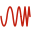# Frequency Options

The supported frequency options are single frequency, continuous range, linearly spaced discrete points, logarithmically spaced discrete points and list of discrete points. Select the frequency option that is best suited to the model and the specified requests.

Note: Frequencies can be specified globally or per configuration.
On the Source/Load tab, in the Settings group, click theFrequency icon.
Single frequency
The requested results are calculated at a single frequency.
Continuous (interpolated) range
The requested results are calculated using adaptive sampling in the range Start frequency to End frequency. The sampling algorithm uses finer sampling in areas where the results change rapidly to ensure that all resonance effects are calculated accurately.
Note: Use this option with as little result requests as possible, since the requested results are interpolated and increases the run time.
Linearly spaced discrete points
The requested results are calculated at a fixed number of linearly spaced points between the Start frequency and the End frequency. This option is typically used when the solution is required at exact frequencies.
Logarithmically spaced discrete points
The requested results are calculated at a fixed number of logarithmically spaced points between the Start frequency and the End frequency. This is typically used over a wide bandwidth.
List of discrete points
The requested results are calculated at a list of discrete points. This is typically used when the exact frequencies are known where the solution is required.
Tip: Use point entry (Ctrl+Shift+left click) to set the frequency to a defined variable in the model tree.